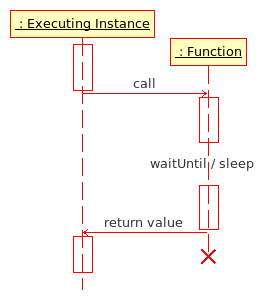# Function

A function is a piece of code which performs performs a specific task and is relatively indepent of the remaining code. They often accept input parameters and sometimes return values back to the script that called them.

## Introduction

Functions were first introduced in the OFP: Resistance patch.

## Usage

Functions should be used for any processes where the result or calculation done in the function is important. This result or calculation should be made in the least time possible. They are different to scripts, where timing is important.

Functions can also be used to reuse code. You can write some code once in the function and then include it in many different scripts. When the code is updated, it is updated for all scripts. When you only copy and paste the code to the other scripts, you have to update every script on any change.

## Syntax

Functions are strictly limited to SQF syntax.

## ExecutionFunction Execution Diagram

Executing Instance: script, function or game engine

Functions can be executed from several points in the game:

Functions are first loaded as String from a file via preprocessFile or loadFile. They are then executed via the call command. Since Armed Assault the loaded String needs to be compiled in order to convert it to Code, which is requried for call.

Example (Operation Flashpoint):

```myFunction1 = loadFile "myFunction1.sqf";
myFunction2 = preprocessFile "myFunction2.sqf";

call myFunction1;
[1, 2] call myFunction2;
```

Example (Armed Assault):

```myFunction1 = compile loadFile "myFunction1.sqf";
myFunction2 = compile preprocessFile "myFunction2.sqf";

call myFunction1;
[1, 2] call myFunction2;
```

Functions are running within the executing instance, which waits for the result of the function. Different to scripts, functions halt all other game engine processes until the function has completed its instructions. This means functions run faster than scripts, and the result of functions is immediate and unambiguous. It can also mean that if a function takes too long to run it will have an adverse effect on game play - large functions or CPU intensive functions can cause the game to seize up until it completes. When creating a functions you want the function to be short and sweet to achieve the best results.

Note: You can still use the special variables and commands of scripts in functions (Armed Assault only)!

## Limitations

Functions have a limitation of 10,000 loops before they are forced to exit by the game engine in order to maintain some level of stability.

## Return Value

The last expression given in a function is returned to the calling instance. Note that there must not be a semicolon after this value.

return.sqf

```STATEMENT 1;
STATEMENT 2;
RETURN_VALUE
```

test.sqf

```value = call compile preprocessFile "return.sqf";
// value is now RETURN_VALUE

call compile preprocessFile "return.sqf";
// valid, but RETURN_VALUE is not saved anywhere
```

## Examples

### Example 1: max.sqf

In this example the function returns maximum of first and second argument.

max.sqf

```comment "Return maximum of first and second argument";
private ["_a","_b"];
_a = _this select 0;
_b = _this select 1;
if (_a>_b) then {_a} else {_b}
```

executing script:

```fMax = compile preprocessFile "max.sqf";
maxValue = [3,5] call fMax;

// maxValue is now 5
```

### Example 2: infantrySafe.sqf

In this example the function returns no value and switches all units to safe mode.

```comment "Switch all infantry units to safe mode";
{
if (vehicle _x == _x) then
{
_x setBehaviour "safe"
}
} forEach _this
```

### Example 3: Inline Function

An inline-function can be created in any script:

```FNC_sayhello = {hint format["hello %1",_this]};
```

This function can then be called (in other scripts, functions, unit's init lines, trigger activation fields, etc.) via:

```name player call FNC_sayhello
```

Notice that there are no brackets around the functions arguments which precede the call command.
In case the function doesn't require any arguments you can just call the function.

```call FNC_helloall
```